# Visualizing epoched data#

This tutorial shows how to plot epoched data as time series, how to plot the spectral density of epoched data, how to plot epochs as an imagemap, and how to plot the sensor locations and projectors stored in `Epochs` objects.

We’ll start by importing the modules we need, loading the continuous (raw) sample data, and cropping it to save memory:

```import mne

sample_data_folder = mne.datasets.sample.data_path()
sample_data_raw_file = (sample_data_folder / 'MEG' / 'sample' /
'sample_audvis_raw.fif')
```

To create the `Epochs` data structure, we’ll extract the event IDs stored in the stim channel, map those integer event IDs to more descriptive condition labels using an event dictionary, and pass those to the `Epochs` constructor, along with the `Raw` data and the desired temporal limits of our epochs, `tmin` and `tmax` (for a detailed explanation of these steps, see The Epochs data structure: discontinuous data).

```events = mne.find_events(raw, stim_channel='STI 014')
event_dict = {'auditory/left': 1, 'auditory/right': 2, 'visual/left': 3,
'visual/right': 4, 'face': 5, 'button': 32}
epochs = mne.Epochs(raw, events, tmin=-0.2, tmax=0.5, event_id=event_dict,
del raw
```
```176 events found
Event IDs: [ 1  2  3  4  5 32]
176 matching events found
Setting baseline interval to [-0.19979521315838786, 0.0] sec
Applying baseline correction (mode: mean)
Created an SSP operator (subspace dimension = 3)
3 projection items activated
```

## Plotting `Epochs` as time series#

To visualize epoched data as time series (one time series per channel), the `mne.Epochs.plot` method is available. It creates an interactive window where you can scroll through epochs and channels, enable/disable any unapplied SSP projectors to see how they affect the signal, and even manually mark bad channels (by clicking the channel name) or bad epochs (by clicking the data) for later dropping. Channels marked “bad” will be shown in light grey color and will be added to `epochs.info['bads']`; epochs marked as bad will be indicated as `'USER'` in `epochs.drop_log`.

Here we’ll plot only the “catch” trials from the sample dataset, and pass in our events array so that the button press responses also get marked (we’ll plot them in red, and plot the “face” events defining time zero for each epoch in blue). We also need to pass in our `event_dict` so that the `plot` method will know what we mean by “button” — this is because subsetting the conditions by calling `epochs['face']` automatically purges the dropped entries from `epochs.event_id`:

```catch_trials_and_buttonpresses = mne.pick_events(events, include=[5, 32])
epochs['face'].plot(events=catch_trials_and_buttonpresses, event_id=event_dict,
event_color=dict(button='red', face='blue'))
```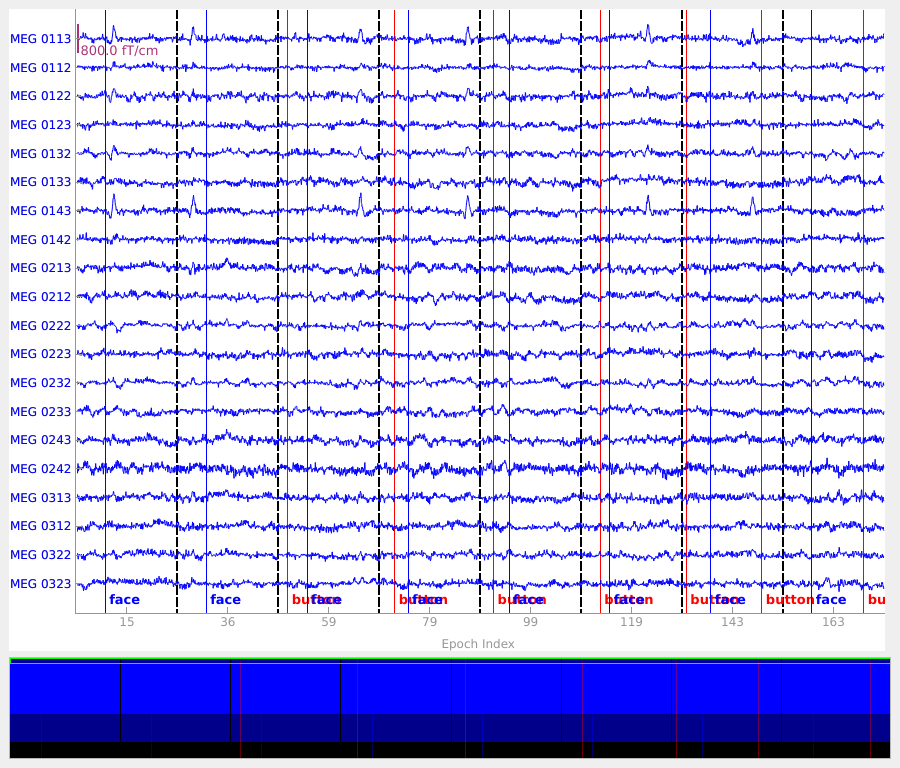To see all sensors at once, we can use butterfly mode and group by selection:

```epochs['face'].plot(events=catch_trials_and_buttonpresses, event_id=event_dict,
event_color=dict(button='red', face='blue'),
group_by='selection', butterfly=True)
```
•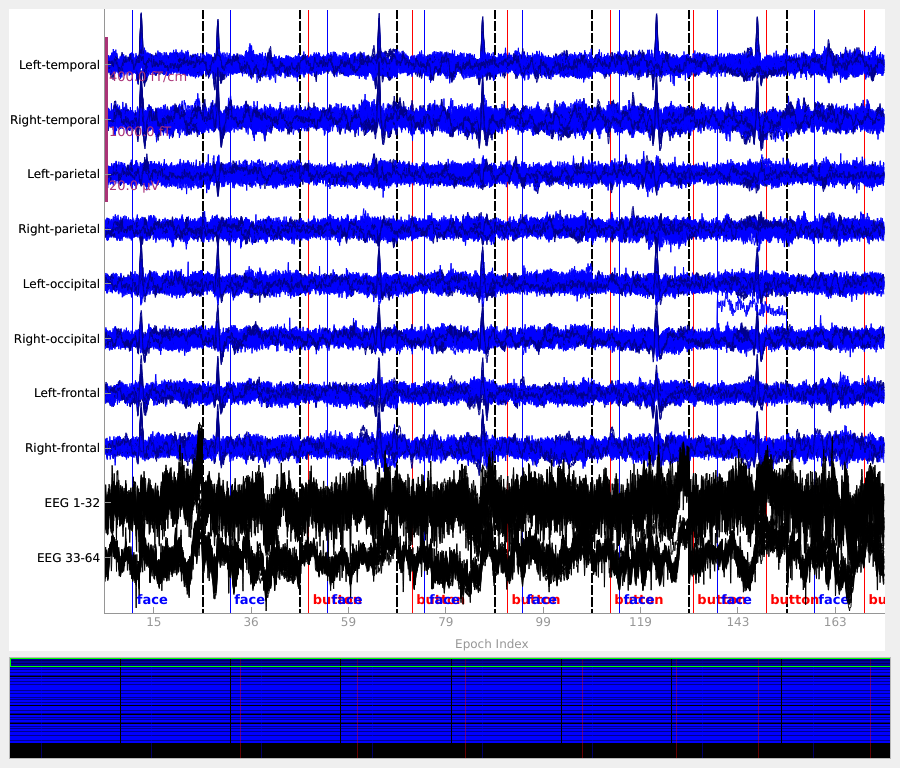•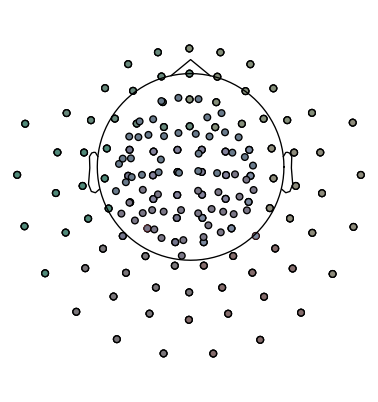## Plotting projectors from an `Epochs` object#

In the plot above we can see heartbeat artifacts in the magnetometer channels, so before we continue let’s load ECG projectors from disk and apply them to the data:

```ecg_proj_file = (sample_data_folder / 'MEG' / 'sample' /
'sample_audvis_ecg-proj.fif')
epochs.apply_proj()
```
```    Read a total of 6 projection items:
ECG-planar-999--0.200-0.400-PCA-01 (1 x 203)  idle
ECG-planar-999--0.200-0.400-PCA-02 (1 x 203)  idle
ECG-axial-999--0.200-0.400-PCA-01 (1 x 102)  idle
ECG-axial-999--0.200-0.400-PCA-02 (1 x 102)  idle
ECG-eeg-999--0.200-0.400-PCA-01 (1 x 59)  idle
ECG-eeg-999--0.200-0.400-PCA-02 (1 x 59)  idle
6 projection items deactivated
Created an SSP operator (subspace dimension = 9)
9 projection items activated
SSP projectors applied...
```
Number of events 175 auditory/left: 41auditory/right: 40button: 8face: 8visual/left: 40visual/right: 38 -0.200 – 0.499 sec -0.200 – 0.000 sec

Just as we saw in the Plotting projectors from Raw objects section, we can plot the projectors present in an `Epochs` object using the same `plot_projs_topomap` method. Since the original three empty-room magnetometer projectors were inherited from the `Raw` file, and we added two ECG projectors for each sensor type, we should see nine projector topomaps:

```epochs.plot_projs_topomap(vlim='joint')
```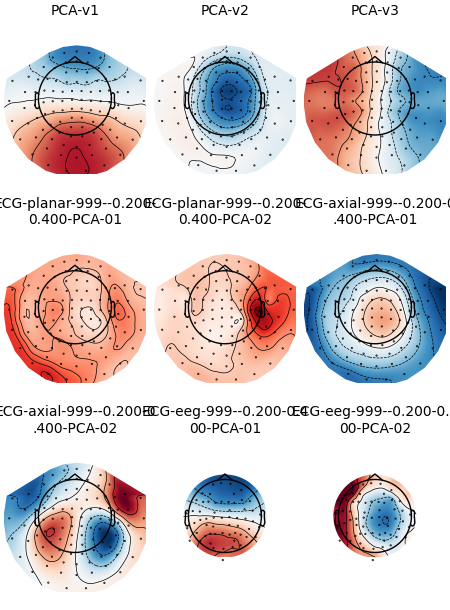Note that these field maps illustrate aspects of the signal that have already been removed (because projectors in `Raw` data are applied by default when epoching, and because we called `apply_proj` after adding additional ECG projectors from file). You can check this by examining the `'active'` field of the projectors:

```print(all(proj['active'] for proj in epochs.info['projs']))
```
```True
```

## Plotting sensor locations#

Just like `Raw` objects, `Epochs` objects keep track of sensor locations, which can be visualized with the `plot_sensors` method:

```epochs.plot_sensors(kind='3d', ch_type='all')
epochs.plot_sensors(kind='topomap', ch_type='all')
```
•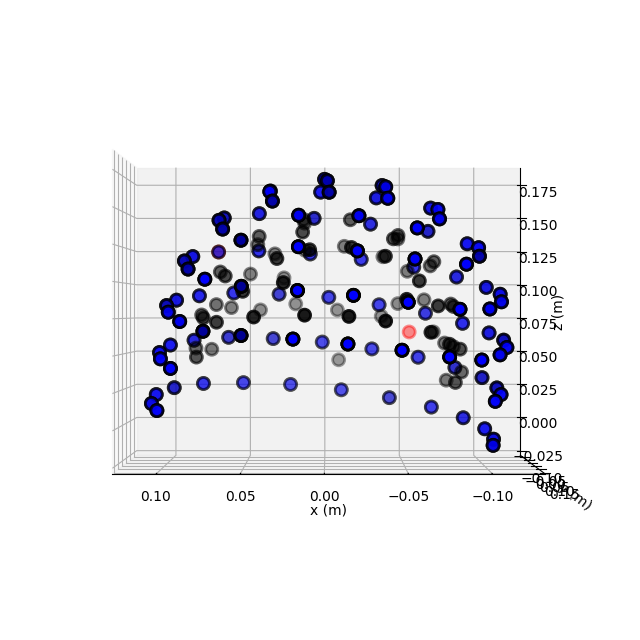•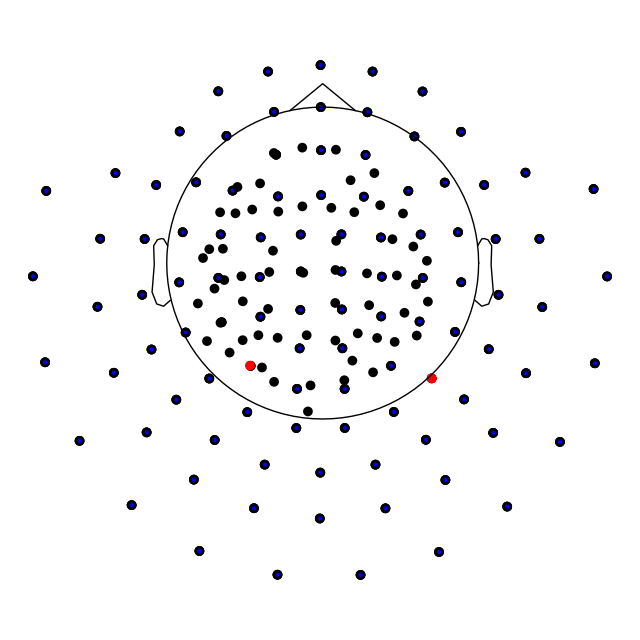## Plotting the power spectrum of `Epochs`#

Again, just like `Raw` objects, `Epochs` objects can be converted to spectral density via `compute_psd()`, which can then be plotted using the `EpochsSpectrum`’s `plot()` method.

```epochs['auditory'].compute_psd().plot(picks='eeg')
```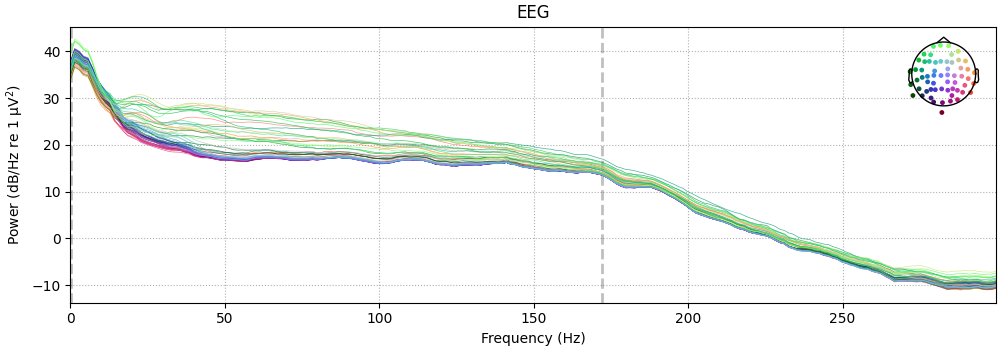```    Using multitaper spectrum estimation with 7 DPSS windows
Averaging across epochs...
```

It is also possible to plot spectral power estimates across sensors as a scalp topography, using the `EpochsSpectrum`’s `plot_topomap()` method. The default parameters will plot five frequency bands (δ, θ, α, β, γ), will compute power based on magnetometer channels (if present), and will plot the power estimates on a dB-like log-scale:

```spectrum = epochs['visual/right'].compute_psd()
spectrum.plot_topomap()
```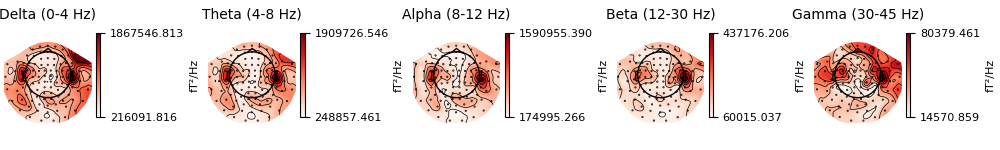```Using multitaper spectrum estimation with 7 DPSS windows
```

Note

Prior to the addition of the `EpochsSpectrum` class, the above plots were possible via:

```epochs['auditory'].plot_psd(picks='eeg')
epochs['visual/right'].plot_psd_topomap()
```

The `plot_psd()` and `plot_psd_topomap` methods of `Epochs` objects are still provided to support legacy analysis scripts, but new code should instead use the `EpochsSpectrum` object API.

Just like `plot_projs_topomap`, `EpochsSpectrum.plot_topomap()` has a `vlim='joint'` option for fixing the colorbar limits jointly across all subplots, to give a better sense of the relative magnitude in each frequency band. You can change which channel type is used via the `ch_type` parameter, and if you want to view different frequency bands than the defaults, the `bands` parameter takes a `dict`, with keys providing a subplot title and values providing either single frequency bins to plot, or lower/upper frequency band edges:

```bands = {'10 Hz': 10, '15 Hz': 15, '20 Hz': 20, '10-20 Hz': (10, 20)}
```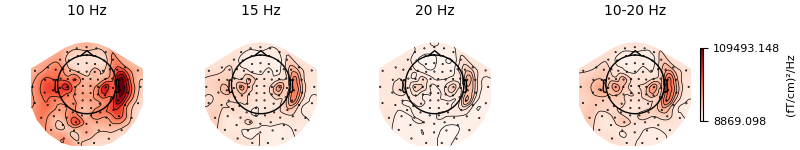If you prefer untransformed power estimates, you can pass `dB=False`. It is also possible to normalize the power estimates by dividing by the total power across all frequencies, by passing `normalize=True`. See the docstring of `plot_topomap` for details.

## Plotting `Epochs` as an image map#

A convenient way to visualize many epochs simultaneously is to plot them as an image map, with each row of pixels in the image representing a single epoch, the horizontal axis representing time, and each pixel’s color representing the signal value at that time sample for that epoch. Of course, this requires either a separate image map for each channel, or some way of combining information across channels. The latter is possible using the `plot_image` method; the former can be achieved with the `plot_image` method (one channel at a time) or with the `plot_topo_image` method (all sensors at once).

By default, the image map generated by `plot_image` will be accompanied by a scalebar indicating the range of the colormap, and a time series showing the average signal across epochs and a bootstrapped 95% confidence band around the mean. `plot_image` is a highly customizable method with many parameters, including customization of the auxiliary colorbar and averaged time series subplots. See the docstrings of `plot_image` and `mne.viz.plot_compare_evokeds` (which is used to plot the average time series) for full details. Here we’ll show the mean across magnetometers for all epochs with an auditory stimulus:

```epochs['auditory'].plot_image(picks='mag', combine='mean')
```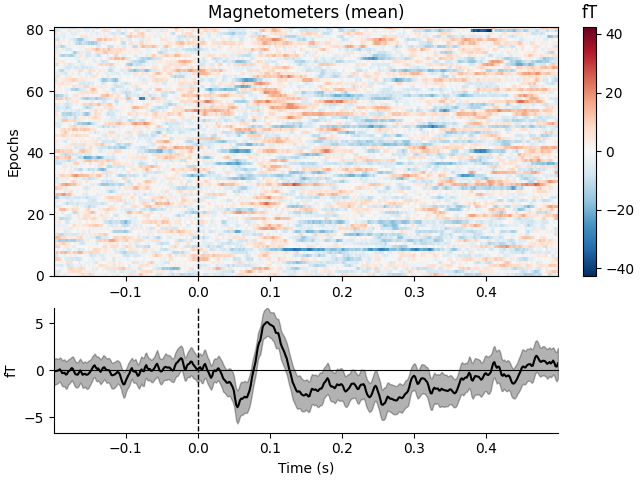```Not setting metadata
81 matching events found
No baseline correction applied
0 projection items activated
combining channels using "mean"
```

To plot image maps for individual sensors or a small group of sensors, use the `picks` parameter. Passing `combine=None` (the default) will yield separate plots for each sensor in `picks`; passing `combine='gfp'` will plot the global field power (useful for combining sensors that respond with opposite polarity).

```epochs['auditory'].plot_image(picks=['MEG 0242', 'MEG 0243'])
epochs['auditory'].plot_image(picks=['MEG 0242', 'MEG 0243'], combine='gfp')
```
•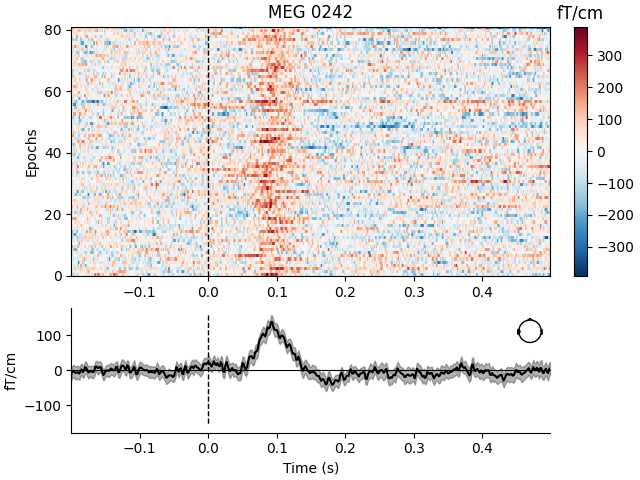•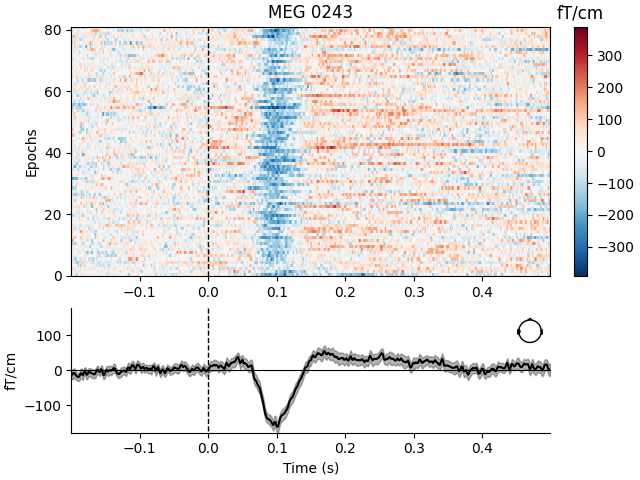•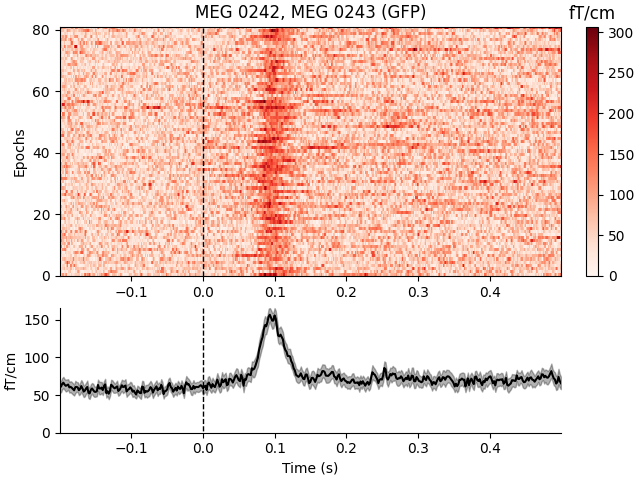```Not setting metadata
81 matching events found
No baseline correction applied
0 projection items activated
81 matching events found
No baseline correction applied
0 projection items activated
81 matching events found
No baseline correction applied
0 projection items activated
combining channels using "gfp"
```

To plot an image map for all sensors, use `plot_topo_image`, which is optimized for plotting a large number of image maps simultaneously, and (in interactive sessions) allows you to click on each small image map to pop open a separate figure with the full-sized image plot (as if you had called `plot_image` on just that sensor). At the small scale shown in this tutorial it’s hard to see much useful detail in these plots; it’s often best when plotting interactively to maximize the topo image plots to fullscreen. The default is a figure with black background, so here we specify a white background and black foreground text. By default `plot_topo_image` will show magnetometers and gradiometers on the same plot (and hence not show a colorbar, since the sensors are on different scales) so we’ll also pass a `Layout` restricting each plot to one channel type. First, however, we’ll also drop any epochs that have unusually high signal levels, because they can cause the colormap limits to be too extreme and therefore mask smaller signal fluctuations of interest.

```reject_criteria = dict(mag=3000e-15,     # 3000 fT
eeg=150e-6)       # 150 µV

layout = mne.channels.find_layout(epochs.info, ch_type=ch_type)
epochs['auditory/left'].plot_topo_image(layout=layout, fig_facecolor='w',
font_color='k', title=title)
```
•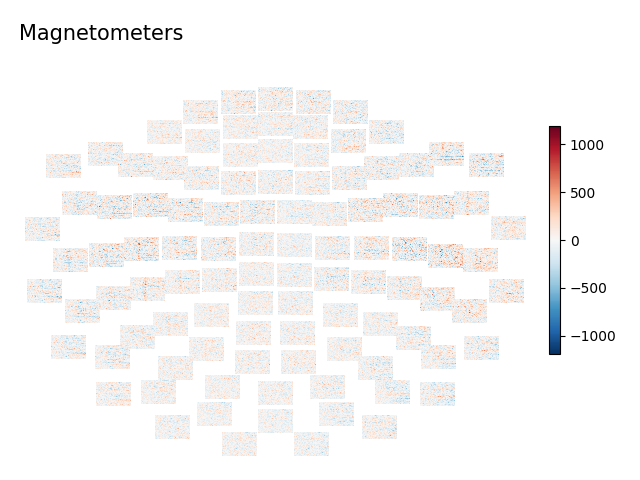•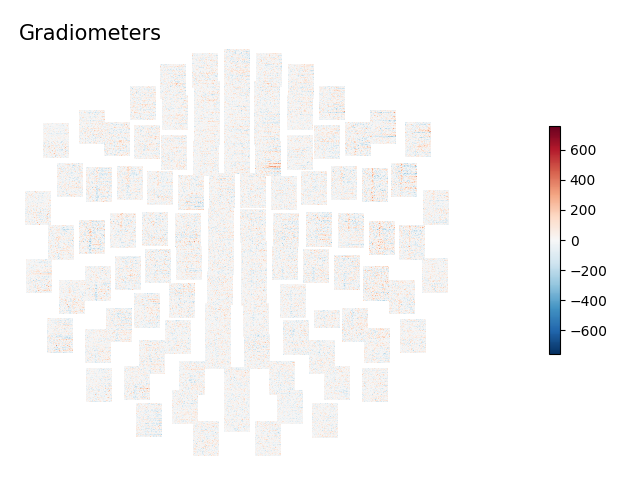```    Rejecting  epoch based on EEG : ['EEG 001', 'EEG 002', 'EEG 003', 'EEG 004', 'EEG 005', 'EEG 006', 'EEG 007', 'EEG 015', 'EEG 016', 'EEG 023', 'EEG 039', 'EEG 041', 'EEG 044', 'EEG 045', 'EEG 046', 'EEG 047', 'EEG 048', 'EEG 049', 'EEG 050', 'EEG 051', 'EEG 052', 'EEG 054', 'EEG 055', 'EEG 056', 'EEG 057', 'EEG 058', 'EEG 059']
Rejecting  epoch based on EEG : ['EEG 001', 'EEG 002', 'EEG 003', 'EEG 007', 'EEG 048', 'EEG 055']
Rejecting  epoch based on EEG : ['EEG 007']
Rejecting  epoch based on EEG : ['EEG 003', 'EEG 007']
Rejecting  epoch based on MAG : ['MEG 1711']
Rejecting  epoch based on EEG : ['EEG 001', 'EEG 002', 'EEG 003', 'EEG 007']
Rejecting  epoch based on EEG : ['EEG 001', 'EEG 002', 'EEG 007']
Rejecting  epoch based on MAG : ['MEG 1711']
Removing projector <Projection | ECG-planar-999--0.200-0.400-PCA-01, active : True, n_channels : 203>
Removing projector <Projection | ECG-planar-999--0.200-0.400-PCA-02, active : True, n_channels : 203>
Removing projector <Projection | ECG-eeg-999--0.200-0.400-PCA-01, active : True, n_channels : 59>
Removing projector <Projection | ECG-eeg-999--0.200-0.400-PCA-02, active : True, n_channels : 59>
Removing projector <Projection | PCA-v1, active : True, n_channels : 102>
Removing projector <Projection | PCA-v2, active : True, n_channels : 102>
Removing projector <Projection | PCA-v3, active : True, n_channels : 102>
Removing projector <Projection | ECG-axial-999--0.200-0.400-PCA-01, active : True, n_channels : 102>
Removing projector <Projection | ECG-axial-999--0.200-0.400-PCA-02, active : True, n_channels : 102>
Removing projector <Projection | ECG-eeg-999--0.200-0.400-PCA-01, active : True, n_channels : 59>
Removing projector <Projection | ECG-eeg-999--0.200-0.400-PCA-02, active : True, n_channels : 59>
```

To plot image maps for all EEG sensors, pass an EEG layout as the `layout` parameter of `plot_topo_image`. Note also here the use of the `sigma` parameter, which smooths each image map along the vertical dimension (across epochs) which can make it easier to see patterns across the small image maps (by smearing noisy epochs onto their neighbors, while reinforcing parts of the image where adjacent epochs are similar). However, `sigma` can also disguise epochs that have persistent extreme values and maybe should have been excluded, so it should be used with caution.

```layout = mne.channels.find_layout(epochs.info, ch_type='eeg')
epochs['auditory/left'].plot_topo_image(layout=layout, fig_facecolor='w',
font_color='k', sigma=1)
```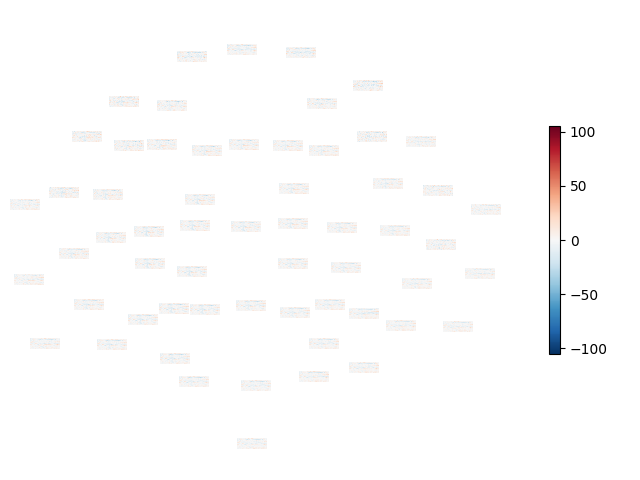```Removing projector <Projection | PCA-v1, active : True, n_channels : 102>
Removing projector <Projection | PCA-v2, active : True, n_channels : 102>
Removing projector <Projection | PCA-v3, active : True, n_channels : 102>
Removing projector <Projection | ECG-planar-999--0.200-0.400-PCA-01, active : True, n_channels : 203>
Removing projector <Projection | ECG-planar-999--0.200-0.400-PCA-02, active : True, n_channels : 203>
Removing projector <Projection | ECG-axial-999--0.200-0.400-PCA-01, active : True, n_channels : 102>
Removing projector <Projection | ECG-axial-999--0.200-0.400-PCA-02, active : True, n_channels : 102>
```

Total running time of the script: ( 0 minutes 35.643 seconds)

Estimated memory usage: 595 MB

Gallery generated by Sphinx-Gallery